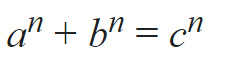# An interesting work with numbers

So why are you giving the example that you want to use 12?

2 Likes

Only for radians; you can do this with degrees between -57 to 57.

It looks like the calculator is broken; in no way should arccosine return a square root.

1 Like

Hello, please tell me other topics. I want an interesting idea to be done with numbers that is unique in the world.

Find the square root of -1

2 Likes

Resolve `Pi`

1 Like

I left this one, of course you mean the number Pi, please another one ؟

Code the Fourier Transform in blocks only.
Input:

• duration in ms
• list of readings over that duration
1 Like

I left this

Monsieur Pierre de Fermat is looking for three integers a, b, c that satisfy the equation:for any integer value of n greater than 2.

2 Likes

Finding prime numbers by method given by a Greek mathematician, Eratosthenes of Alexandria.

1 Like

And,

How can we draw a line whose length is equal to square root of 2.

1 Like

That's interesting, but the square root of 2 is about 1.4. You might want to draw a line which length is √2 × 100 pixels.

1 Like

I did something like this:

As you can see, there is a yellow, blue and white line. The white line separates the phone screen into 2 equal parts. If the width of each part is 1, then the blue line is √2.

Now, if you ignore the blue line and the white line, there is also a yellow line. The yellow line is √2 ✕ 100 pixels long.

You could also check Proposals of math codes. Area polygon. Vertices rectangle. Linear regression. Decimal IP topic. There are some unfinished projects that you could work with

4 Likes